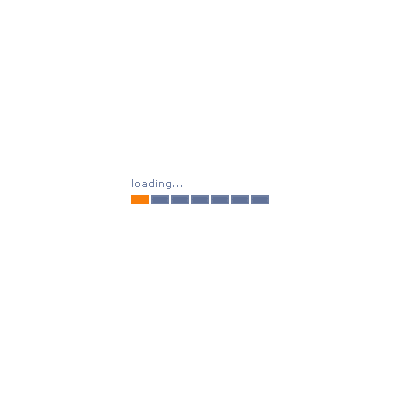Whie是最基本的循环，它的结构为:

``````whil (布尔表达式) {
// 循环体语句。
}``````EG:

``````package com.zctou.structure;

public class WhileDemo01 {
public static void main(String[] args) {
//输出1-100
int i = 0 ;
while (i<=100) {
System.out.println(i);
i++ ;
}
}
}
``````

``````0
1
2
...
99
100``````

EG2: 计算1+2+...+100的结果：

``````package com.zctou.structure;

public class WhileDeomo02 {
public static void main(String[] args) {
//计算1+2+...+100的结果：

int i = 0 ;
int sum = 0 ;

while (i<=100) {
sum = sum+ i ;
i++ ;
}
System.out.println(sum);
}
}
``````

``5050``